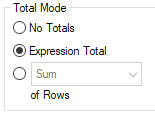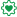# New to Qlik Sense

Discussion board where members can get started with Qlik Sense.

Announcements
Talk to Experts, a LIVE Q&A Webinar. Bring your Qlik Sense Business questions on Aug. 4th. Register
cancel
Showing results for
Did you mean:
HighlightedCreator II

## Old Topic: difference sum and the qualifier TOTAL

Hello,

I got an old topic where someone tried to explain the difference between the aggregation sum and the qualifier TOTAL.

Can someone explain to me in plain words what he meant?

Here is the old post:

Re: Difference between sum and expression Total in Straight table

Hi,

Sum - will give you the sum of all rows in the table

Expression Total - will give you the overall expression total.

For example:

Year     A     B

2013     50     100

2014     50     150

When you use A/B as expression and use Year as Dimension

Sum Mode - 83% (50 + 33) (50/100 + 50/150 = 50% + 33 % = 83%)

Expression Mode - 40% (100/250) (50 + 50) / (100 + 150)

I understand fully the explanation in the Qlik Website:

Year Quarter Sum(Amount) Sum({1} TOTAL Amount) Sum(Amount)/Sum({1} TOTAL Amount)
 3000 10800 27,8% 2012 Q2 1700 10800 15,7% 2013 Q2 1300 10800 12%

But there is also only ONE Column of Amount to calculate.

Can someone please explain me his calculation in plain words (purple highlighted)?

Thank you!

1 Solution

Accepted Solutions
HighlightedMVP & Luminary

## Re: Old Topic: difference sum and the qualifier TOTAL

The explanation in purple is not about the TOTAL qualifier. The explanation is about the "Total Mode" property available in QlikView Straight Table, but not in Qlik Sense.  It controls how the Total row in the chart is calculated.-Rob

3 Replies
HighlightedEmployee

## Re: Old Topic: difference sum and the qualifier TOTAL

The simple explanation is that the TOTAL qualifier ignores dimensions and calculates the measure across all dimensions.

HighlightedMVP & Luminary

## Re: Old Topic: difference sum and the qualifier TOTAL

The explanation in purple is not about the TOTAL qualifier. The explanation is about the "Total Mode" property available in QlikView Straight Table, but not in Qlik Sense.  It controls how the Total row in the chart is calculated.-Rob

HighlightedPartner